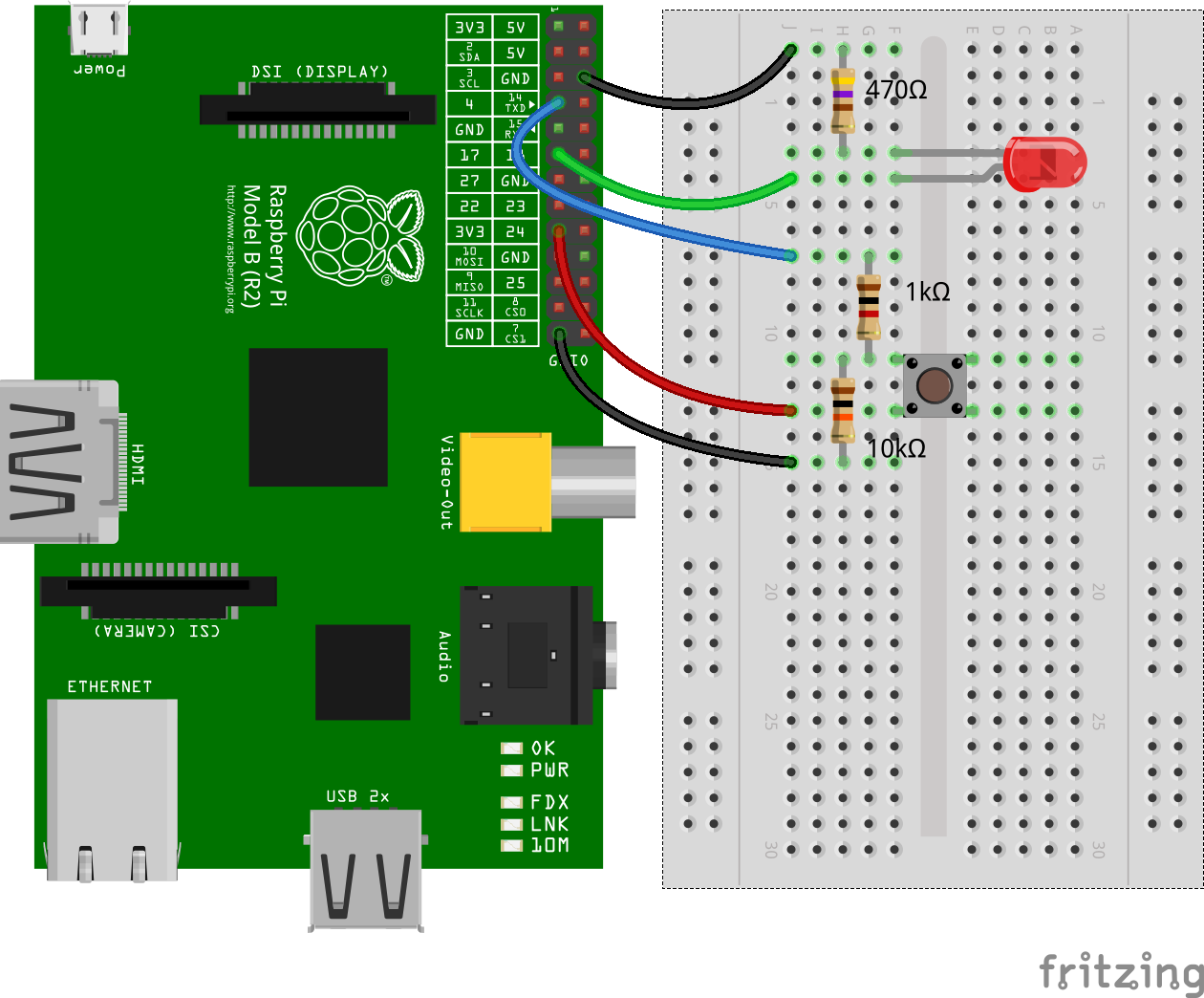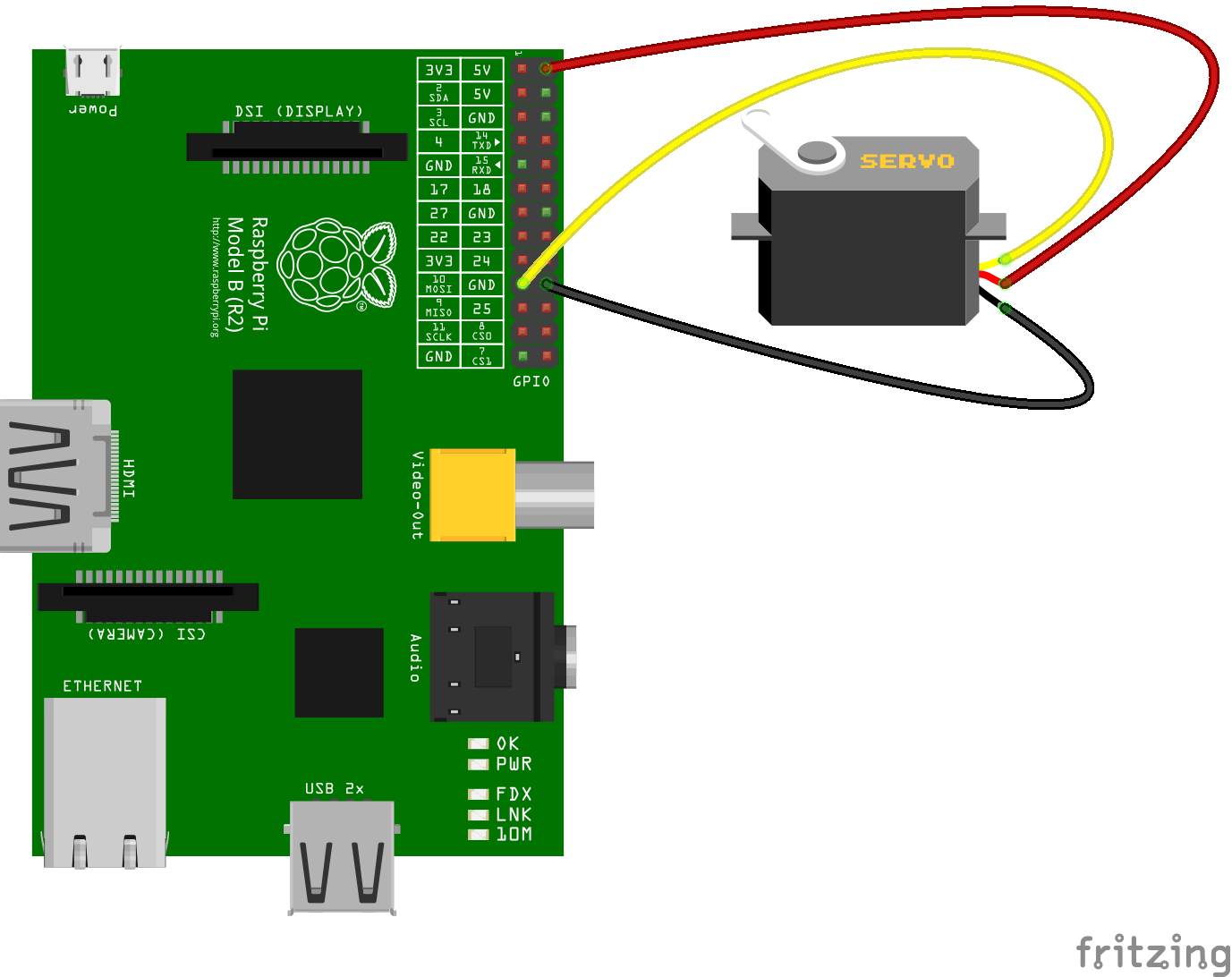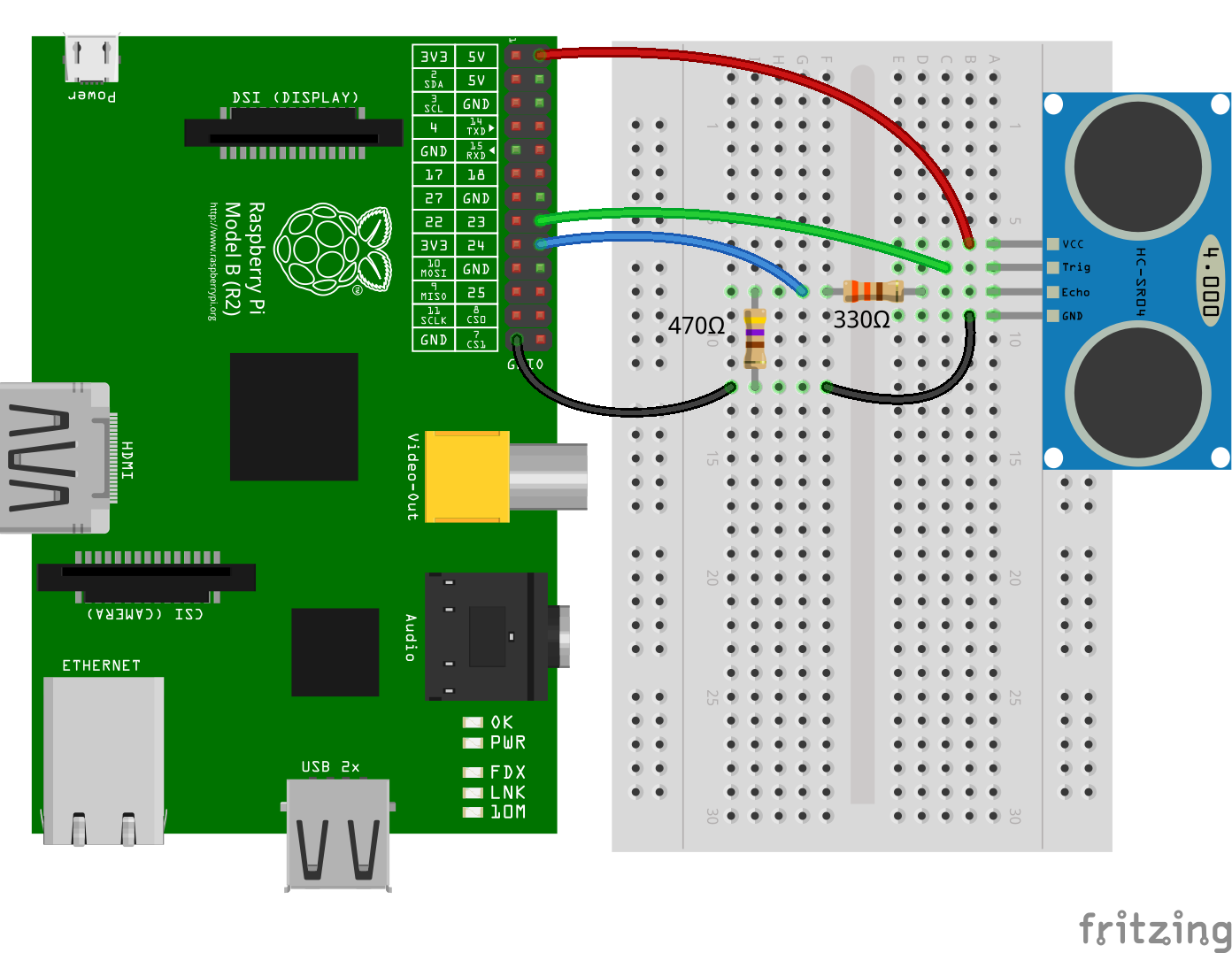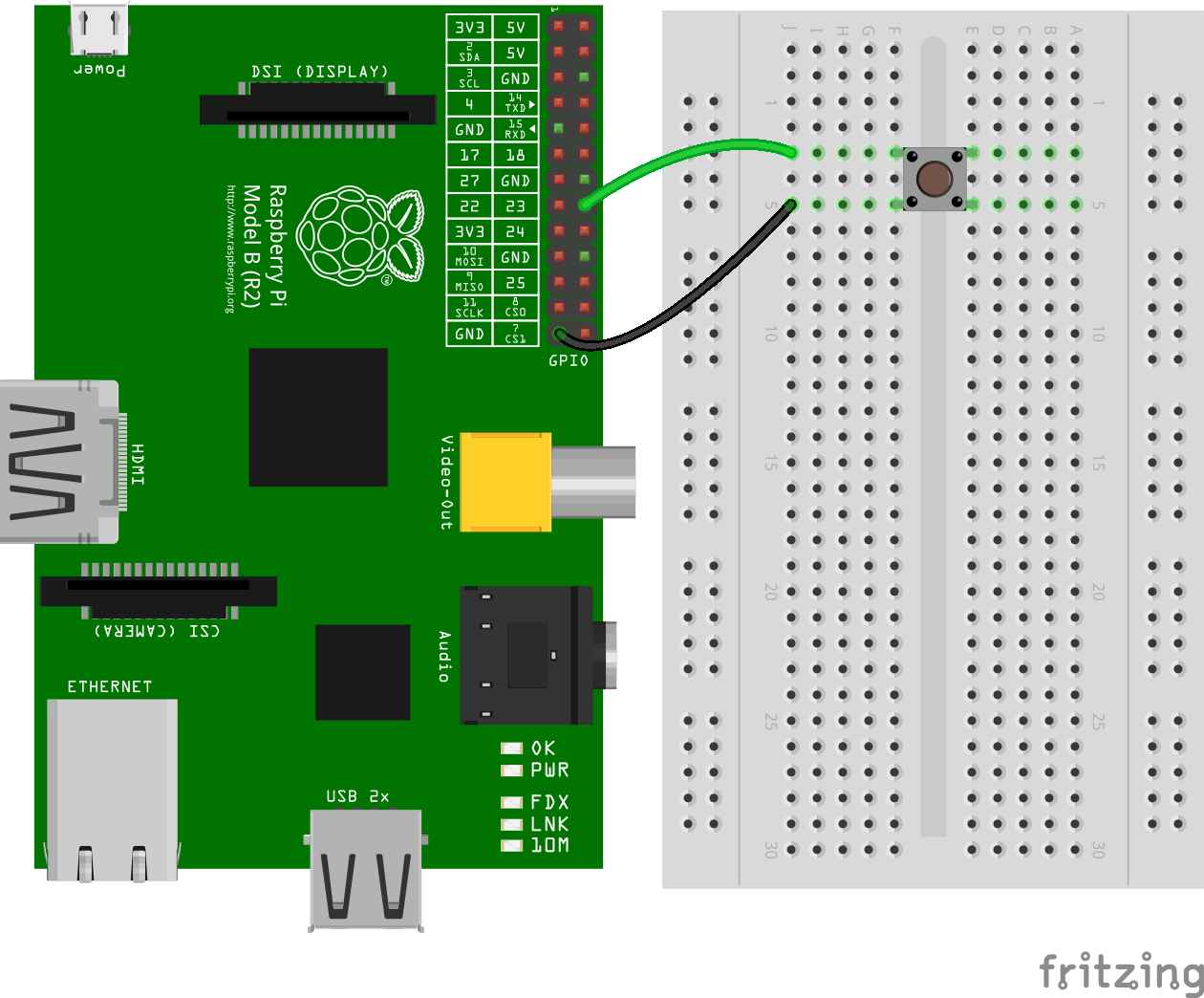# pigpio

A wrapper for the pigpio C library to enable fast GPIO, PWM, servo control, state change notification and interrupt handling with Node.js on the Raspberry Pi Zero, 1, 2, 3 or 4.

pigpio supports Node.js versions 10, 12, 14, 15 and 16.

## Features

• Digital IO
• Up to 3.5 million digital reads per second *)
• Up to 2.5 million digital writes per second *)
• PWM on any of GPIOs 0 through 31
• Multiple frequencies and duty cycle ranges supported
• Servo control on any of GPIOs 0 through 31
• Jitter free
• Alerts when any of GPIOs 0 through 31 change state
• The time of the state change is available accurate to a few microseconds
• Notification streams for monitoring state changes on any of GPIOs 0 through 31 concurrently
• The time of the state changes are available accurate to a few microseconds
• Low latency interrupt handlers
• Handle up to 20000 interrupts per second *)
• Read or write up to 32 GPIOs as one operation with banked GPIO
• Trigger pulse generation
• Pull up/down resistor configuration
• Waveforms to generate GPIO level changes (time accurate to a few µs)

*) On a Raspberry Pi 4 Model B running Raspberry Pi OS 2021-03-04 (Buster 10.8) with pigpio v3.3.1, Node.js v16.0.0 and V79 of the pigpio C library.

## Installation

#### Step 1 - Install the pigpio C library

The pigpio C library is a prerequisite for the pigpio Node.js module.

Run the following command to determine which version of the pigpio C library is installed:

``````pigpiod -v
``````

For the Raspberry Pi Zero, 1, 2 and 3 V41 or higher of the pigpio C library is required. For the Raspberry Pi 4 V69 or higher is required.

If the pigpio C library is not installed or if the installed version is too old, the latest version can be installed with the following commands:

``````sudo apt-get update
sudo apt-get install pigpio
``````

Alternative installation instructions for the pigpio C library can be found here.

Warning: The pigpio C library contains a number of utilities. One of these utilities is pigpiod which launches the pigpio C library as a daemon. This utility should not be used as the pigpio Node.js package uses the C library directly.

#### Step 2 - Install the pigpio Node.js package

``````npm install pigpio
``````

## Usage

Assume there's an LED connected to GPIO17 (pin 11) and a momentary push button connected to GPIO4 (pin 7).#### Pulse an LED with PWM

Use PWM to pulse the LED connected to GPIO17 from fully off to fully on continuously.

``````const Gpio = require('pigpio').Gpio;

const led = new Gpio(17, {mode: Gpio.OUTPUT});

let dutyCycle = 0;

setInterval(() => {
led.pwmWrite(dutyCycle);

dutyCycle += 5;
if (dutyCycle > 255) {
dutyCycle = 0;
}
}, 20);
``````

#### Buttons and Interrupt Handling

Turn the LED connected to GPIO17 on when the momentary push button connected to GPIO4 is pressed. Turn the LED off when the button is released.

``````const Gpio = require('pigpio').Gpio;

const led = new Gpio(17, {mode: Gpio.OUTPUT});
const button = new Gpio(4, {
mode: Gpio.INPUT,
pullUpDown: Gpio.PUD_DOWN,
edge: Gpio.EITHER_EDGE
});

button.on('interrupt', (level) => {
led.digitalWrite(level);
});
``````

#### Servo Control

Continuously move a servo connected to GPIO10 clockwise and anti-clockwise.``````const Gpio = require('pigpio').Gpio;

const motor = new Gpio(10, {mode: Gpio.OUTPUT});

let pulseWidth = 1000;
let increment = 100;

setInterval(() => {
motor.servoWrite(pulseWidth);

pulseWidth += increment;
if (pulseWidth >= 2000) {
increment = -100;
} else if (pulseWidth <= 1000) {
increment = 100;
}
}, 1000);
``````

#### Measure Distance with a HC-SR04 Ultrasonic Sensor

The `trigger` function can be used to generate a pulse on a GPIO and alerts can be used to determine the time of a GPIO state change accurate to a few microseconds. These two features can be combined to measure distance using a HC-SR04 ultrasonic sensor.``````const Gpio = require('pigpio').Gpio;

// The number of microseconds it takes sound to travel 1cm at 20 degrees celcius
const MICROSECDONDS_PER_CM = 1e6/34321;

const trigger = new Gpio(23, {mode: Gpio.OUTPUT});
const echo = new Gpio(24, {mode: Gpio.INPUT, alert: true});

trigger.digitalWrite(0); // Make sure trigger is low

const watchHCSR04 = () => {
let startTick;

if (level == 1) {
startTick = tick;
} else {
const endTick = tick;
const diff = (endTick >> 0) - (startTick >> 0); // Unsigned 32 bit arithmetic
console.log(diff / 2 / MICROSECDONDS_PER_CM);
}
});
};

watchHCSR04();

// Trigger a distance measurement once per second
setInterval(() => {
trigger.trigger(10, 1); // Set trigger high for 10 microseconds
}, 1000);
``````

#### Determine the Width of a Pulse with Alerts

Alerts can be used to determine the time of a GPIO state change accurate to a few microseconds. Typically, alerts will be used for GPIO inputs but they can also be used for outputs. In this example, the `trigger` method is used to pulse the LED connected to GPIO17 on for 15 microseconds once per second. Alerts are used to measure the length of the pulse.

``````// Assumption: the LED is off when the program is started

const Gpio = require('pigpio').Gpio;

const led = new Gpio(17, {
mode: Gpio.OUTPUT,
});

const watchLed = () => {
let startTick;

// Use alerts to determine how long the LED was turned on
if (level == 1) {
startTick = tick;
} else {
const endTick = tick;
const diff = (endTick >> 0) - (startTick >> 0); // Unsigned 32 bit arithmetic
console.log(diff);
}
});
};

watchLed();

// Turn the LED on for 15 microseconds once per second
setInterval(() => {
led.trigger(15, 1);
}, 1000);
``````

Here's an example of the typical output to the console:

``````15
15
15
15
15
15
20
15
15
15
15
``````

#### Debounce a Button

The GPIO glitch filter will prevent alert events from being emitted if the corresponding level change is not stable for at least a specified number of microseconds. This can be used to filter out unwanted noise from an input signal. In this example, a glitch filter is applied to filter out the contact bounce of a push button.``````const Gpio = require('pigpio').Gpio;

const button = new Gpio(23, {
mode: Gpio.INPUT,
pullUpDown: Gpio.PUD_UP,
});

let count = 0;

// Level must be stable for 10 ms before an alert event is emitted.
button.glitchFilter(10000);

if (level === 0) {
console.log(++count);
}
});
``````

#### Generate a waveform

Waveforms can be used to time and execute Gpio level changes with an accuracy up to 1 microsecond. The following example generates a waveform that starts with a 1µs pulse, then has a 2µs pause, followed by a 3µs pulse and so on. The waveform definition is a simple Array where each entry is an object with the properties gpioOn, gpioOff and usDelay.

The basic workflow to generate and execute waveforms is as follows:

First, we usually clear previous wave entries with the `waveClear` method. Then we can add pulses with the `waveAddGeneric` method to the cleared waveform. We then create a waveId by calling the `waveCreate` method. To execute the waveform, we call the `waveTxSend` method. Once the wave is sent, we can delete the wave by calling the `waveDelete` method.

``````const pigpio = require('pigpio');
const Gpio = pigpio.Gpio;

const outPin = 17;

const output = new Gpio(outPin, {mode: Gpio.OUTPUT});

output.digitalWrite(0);
pigpio.waveClear();

let waveform = [];

for (let x = 0; x < 20; x++) {
if (x % 2 === 1) {
waveform.push({ gpioOn: outPin, gpioOff: 0, usDelay: x + 1 });
} else {
waveform.push({ gpioOn: 0, gpioOff: outPin, usDelay: x + 1 });
}
}

let waveId = pigpio.waveCreate();

if (waveId >= 0) {
pigpio.waveTxSend(waveId, pigpio.WAVE_MODE_ONE_SHOT);
}

while (pigpio.waveTxBusy()) {}

pigpio.waveDelete(waveId);
``````

#### Sending a wavechain

The `waveChain` method allows you to chain multiple waveforms together. A chain is basically just an array with several waveId's. However you can insert different modifiers as described here.

In the example the `chain` consists of two waves. The first waveform is transmitted normally, then the second waveform is repeated 3 times.

``````const pigpio = require('pigpio');
const Gpio = pigpio.Gpio;

const outPin = 17;
const output = new Gpio(outPin, {mode: Gpio.OUTPUT});

output.digitalWrite(0);
pigpio.waveClear();

let firstWaveForm = [];
let secondWaveForm = [];

for (let x = 0; x < 10; x++) {
if (x % 2 === 0) {
firstWaveForm.push({ gpioOn: outPin, gpioOff: 0, usDelay: 10 });
} else {
firstWaveForm.push({ gpioOn: 0, gpioOff: outPin, usDelay: 10 });
}
}

let firstWaveId = pigpio.waveCreate();

for (let x = 0; x < 10; x++) {
if (x % 2 === 0) {
secondWaveForm.push({ gpioOn: outPin, gpioOff: 0, usDelay: 20 });
} else {
secondWaveForm.push({ gpioOn: 0, gpioOff: outPin, usDelay: 20 });
}
}

let secondWaveId = pigpio.waveCreate();

if (firstWaveId >= 0 && secondWaveId >= 0) {
let chain = [firstWaveId, 255, 0, secondWaveId, 255, 1, 3, 0];
pigpio.waveChain(chain);
}

while (pigpio.waveTxBusy()) {}

pigpio.waveDelete(firstWaveId);
pigpio.waveDelete(secondWaveId);
``````

## API Documentation

### Classes

• Gpio - General Purpose Input Output
• GpioBank - Banked General Purpose Input Output

## Limitations

• The pigpio Node.js package is a wrapper for the pigpio C library. A limitation of the pigpio C library is that it can only be used by a single running process.
• The pigpio C library and therefore the pigpio Node.js package requires root/sudo privileges to access hardware peripherals.

## Troubleshooting

If you have a problem with the library, before you remove it from your code and start trying something else, please check the troubleshooting page first. Some problems are solvable and documented.

## Related Packages

Here are a few links to other hardware specific Node.js packages that may be of interest.

• onoff - GPIO access and interrupt detection
• i2c-bus - I2C serial bus access
• spi-device - SPI serial bus access
• mcp-spi-adc - Analog to digital conversion with the MCP3002/4/8, MCP3202/4/8 and MCP3304
• pigpio-dht - Implements logic to read DHT11 or DHT22/AM2302 temperature and relative humidity sensor
• pigpio-mock - A pigpio mock library for development on your local machine
Alternatives To Pigpio
Select To Compare

Alternative Project Comparisons
Related Awesome Lists

Get A Weekly Email With Trending Projects For These Topics
No Spam. Unsubscribe easily at any time.
Javascript (1,059,387
Raspberry Pi (22,304
Iot (11,916